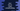# 7 ways to create a LinkedHashMap in Dart## How to create LinkedHashMap in Dart:

There are different ways to create a LinkedHashMap in dart. This post will show you how to create a LinkedHashMap object with examples in dart.

This constructor takes one Map as the parameter and adds the pairs of the map to the LinkedHashMap.

``````import 'dart:collection';

void main() {
final map = <int, String>{1: 'one', 2: 'two', 3: 'three', 4: 'four'};

print('Given map: \${map}');
}``````

Here, map is the given map and hMap is the LinkedHashMap created from this map.

This method takes one Map as its parameter and creates a LinkedHashMap from this map.

Example:

``````import 'dart:collection';

void main() {
final map = <int, String>{1: 'one', 2: 'two', 3: 'three', 4: 'four'};

print('Given map: \${map}');
}``````

It will print:

``````Given map: {1: one, 2: two, 3: three, 4: four}
LinkedHashMap: {1: one, 2: two, 3: three, 4: four}``````

### Method 3: LinkedHashMap.fromEntries(Iterable<MapEntry<K, V>> e)

We can pass a map-entries as the parameter to this method and it creates a LinkedHashMap object.

``````import 'dart:collection';

void main() {
final strList = ['a', 'ab', 'abc', 'abcd', 'abcde'];
final strEntries = strList.map((str) => MapEntry(str.length, str));

print('Given map: \${strList}');
}``````
• The strList is a list of strings.
• We created an iterable of entries by using the map function. It uses the length of the string as its key and the string as its value.
• The LinkedHashMap is created by using the fromEntries method.

If you run this program, it will print:

``````Given map: [a, ab, abc, abcd, abcde]
LinkedHashMap: {1: a, 2: ab, 3: abc, 4: abcd, 5: abcde}``````

### Method 4: LinkedHashMap.fromIterables(Iterable k, Iterable v)

The fromIterables method takes two iterables as its parameters. The content of the first iterable is used as the key of the LinkedHashMap and the content of the second iterable is used as the values of the LinkedHashMap.

``````import 'dart:collection';

void main() {
final intList = [1, 2, 3, 4, 5];
final strList = ['a', 'ab', 'abc', 'abcd', 'abcde'];

}``````

It will print:

``LinkedHashMap: {1: a, 2: ab, 3: abc, 4: abcd, 5: abcde}``

If we try to provide arrays of different lengths, it will throw an error.

For example,

``````import 'dart:collection';

void main() {
final intList = [1, 2, 3, 4, 5, 6, 7];
final strList = ['a', 'ab', 'abc', 'abcd', 'abcde'];

}``````

### Method 5: LinkedHashMap.fromIterable(Iterable it, {K key(dynamic element)?, V value(dynamic element)?})

This constructor takes one iterable and computes the keys and values from the content of the iterable. The key and value calculator functions are optional, if we don’t provide these values, it will use the identify function as default.

``````import 'dart:collection';

void main() {
final strList = ['a', 'ab', 'abc', 'abcd', 'abcde'];

key: (e) => e.length, value: (e) => "_\${e}");

}``````

This function is calculating the keys and values from the iterable strList. The keys are length of each item. Output:

``LinkedHashMap: {1: _a, 2: _ab, 3: _abc, 4: _abcd, 5: _abcde}``

If we don’t provide the second and the third parameter:

``````import 'dart:collection';

void main() {
final strList = ['a', 'ab', 'abc', 'abcd', 'abcde'];

}``````

It will give:

``LinkedHashMap: {a: a, ab: ab, abc: abc, abcd: abcd, abcde: abcde}``

### Method 6: LinkedHashMap({bool equals(K, K)?, int hashCode(K)?, bool isValidKey(dynamic)?})

This is the default constructor. Optionally, it takes three parameters:

• The equals function is used as the comparison function, it uses Object.== by default.
• The hashCode function finds the hash-code of an element. It is Object.hashCode method by default.
• The third parameter isValidKey checks if a key is valid or not.
``````import 'dart:collection';

void main() {
final map = <int, String>{
1: 'one',
2: 'two',
3: 'three',
4: 'four',
5: 'five'
};

equals: (int a, int b) => (b - a) % 3 == 0, hashCode: (int e) => e % 3);

}``````
• It considers two keys as equal if the difference is divisible by 3. The hashcode is equal to the modulo value of the key.

It will print:

``LinkedHashMap: {1: four, 2: five, 3: three}``

Both 1 and 4 are considered equal. So, it updated the key with the second value. Similarly, for 2, it updated the value of 5.

This method creates one identity-based LinkedHashMap.

``````import 'dart:collection';

void main() {
``LinkedHashMap: {}``